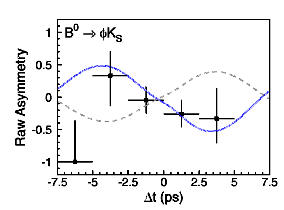# S5

• S5 is an open-source presentation package, written by Eric Meyer.
• Andrea Censi included some basic S5 support in Maruku, his Ruby Markdown implementation.
• My role was to
• make it compatible1 with real XHTML
• integrate it into Instiki
• Driven by Maruku and itex2MML, it’s trivial to include equations, inline SVG graphics, etc.

1. This consisted, mainly, of fixing the Javascript to use DOM-scripting, instead of innerHTML.

# Composing a presentation

An S5 presentation is simply a Wiki page, at the top of which is some metadata:

 author: Jacques Distler
company: University of Texas at Austin
title: S5 Integration
subtitle: slides in Instiki
slide_theme: nautilus
slide_footer: Released to the Internets
slide_subfooter: March 1, 2007

:category: S5-slideshow

The :category: S5-slideshow is essential. It tells Instiki that this page is an S5 slideshow. Any page in this category has an extra “S5” view, in addition to the “TeX” and “Print” views. The other metadata fields are optional. If you omit author: ..., the name of the person who last edited the page is used. If you omit title: ..., the name of the page is used.

# Composing …

After that metadata, comes a series of slides

 My Slideshow
==============

First Slide Title

 * First point
* Second point

Second Slide Title

 * Another boring bullet point.

etc.

Of course, you don’t have to use bullet points. You can use any Markdown (or XHTML) markup you wish.

# Features

1. Mathematics, either inline $\left(\frac{\sin(\pi x)}{\pi x}\right)$ or block
(1)$\int_{\infty}^{\infty}e^{-x^2} \mathrm{d}x = \sqrt{\pi}$

are fully supported. You just type standard itex, and equations like (1) just appear.

2. Incremental display is supported.
3. Notes are allowed. Clicking on (the “Show Notes” control) brings them up in a separate window (on a separate screen, if you have two-screen support). The notes for the current and next slide are visible, along with a presentation timer.

You have to type

 +-- {: .notes}
These are my notes for this slide.
=--

Usually, these optional notes contain the gory details and complicated equations, like $E=m c^2$, too messy to be presented in the main thread of the talk.

# Features II

To get incremental display, use Maruku’s metadata syntax

• Place a {: .incremental .show-first} or {: .incremental} to get incremental display.

• This even works with nested lists.
• Yes, all of S5’s other features are there, too.

• Of course, it doesn’t have all the garish visual effects of Apple’s Keynote.

• If you can’t do without garish, tacky, visual effects, there’s always SVG.

It does have Themes, though. That’s something, isn’t it?

Besides, do you really want to animate

(2)$i \hbar \frac{\partial}{\partial t} \psi = H \psi$

# Features III

Instiki has support for S5 Themes. Themes are stored in the public/s5/themes directory. There are 6 supplied themes:

Choose a theme using the

 slide_theme: NameOfTheme

# Features IVOf course, you can throw in images, like the one at right.

You can, equally well, use inline SVG (at left and below).

$K^0\overline{K}^0$ Mixing

# Features V

Automatic Syntax Coloring:

 require 'chunks/chunk'

# Contains all the methods for finding and replacing wiki links.
module WikiChunk
include Chunk

# A wiki reference is the top-level class for anything that refers to
# another wiki page.
class WikiReference < Chunk::Abstract

# Name of the referenced page

# Name of the referenced page

# the referenced page
def refpage
@content.web.page(@page_name)
end

end

# Features VI

###### Lemma

Let $H$ be a subgroup of a group $G$, and let $x$ and $y$ be elements of $G$. Suppose that $x H \cap y H$ is non-empty. Then $x H = y H$.

###### Proof

Let $z$ be some element of $x H \cap y H$. Then $z = x a$ for some $a \in H$, and $z = y b$ for some $b \in H$. If $h$ is any element of $H$ then $a h \in H$ and $a^{-1}h \in H$, since $H$ is a subgroup of $G$. But $z h = x(a h)$ and $xh = z(a^{-1}h)$ for all $h \in H$. Therefore $z H \subset x H$ and $x H \subset z H$, and thus $x H = z H$. Similarly $y H = z H$, and thus $x H = y H$, as required.

###### Lemma

Let $H$ be a finite subgroup of a group $G$. Then each left coset of $H$ in $G$ has the same number of elements as $H$.

###### Theorem

(Lagrange’s Theorem). Let $G$ be a finite group, and let $H$ be a subgroup of $G$. Then the order of $H$ divides the order of $G$.

# Features VII

You can include Wolfram CDF files, for even more dynamic presentations

# Features VIII

And tables are easy to construct, using itex and Maruku’s extended Markdown table syntax:

$j$$0$$1$$2$$3$$4$$5$$6$$7$$8$
$C\ell_{j}^-$$\mathbb{R}$$\mathbb{C}$$\mathbb{H}$$\mathbb{H}\oplus\mathbb{H}$$\mathbb{H}(2)$$\mathbb{C}(4)$$\mathbb{R}(8)$$\mathbb{R}(8)\oplus\mathbb{R}(8)$$\mathbb{R}(16)$
$C\ell_{j}^+$$\mathbb{R}$$\mathbb{R}\oplus\mathbb{R}$$\mathbb{R}(2)$$\mathbb{C}(2)$$\mathbb{H}(2)$$\mathbb{H}(2)\oplus\mathbb{H}(2)$$\mathbb{H}(4)$$\mathbb{C}(8)$$\mathbb{R}(16)$

Go wild!

If you haven’t already done so, check out the slide controls at the lower right of this slide. Click on to bring up the slide notes (in a separate window). Run through the slide show, perusing the notes.

Look at the regular XHTML view of this slide show (or the Source view). Then head on over to the S5 site to learn more about using S5.